Custom SearchHow the Parallel-LC Circuit Stores Energy A parallel-LC circuit is often called a TANK CIRCUIT because it can store energy much as a tank stores liquid. It has the ability to take energy fed to it from a power source, store this energy alternately in the inductor and capacitor, and produce an output which is a continuous a.c. wave. You can understand how this is accomplished by carefully studying the sequence of events shown in figure 1-8. You must thoroughly understand the capacitor and inductor action in this figure before you proceed further in the study of parallel-resonant circuits. In each view of figure 1-8, the waveform is of the charging and discharging CAPACITOR VOLTAGE. In view (A), the switch has been moved to position C. The d.c. voltage is applied across the capacitor, and the capacitor charges to the potential of the battery. Figure 1-8A. - Capacitor and inductor action in a tank circuit.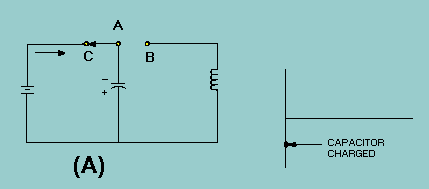In view (B), moving the switch to the right completes the circuit from the capacitor to the inductor and places the inductor in series with the capacitor. This furnishes a path for the excess electrons on the upper plate of the capacitor to flow to the lower plate, and thus starts neutralizing the capacitor charge. As these electrons flow through the coil, a magnetic field is built up around the coil. The energy which was first stored by the electrostatic field of the capacitor is now stored in the electromagnetic field of the inductor. Figure 1-8B. - Capacitor and inductor action in a tank circuit.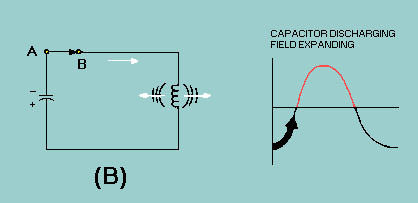View (C) shows the capacitor discharged and a maximum magnetic field around the coil. The energy originally stored in the capacitor is now stored entirely in the magnetic field of the coil. Figure 1-8C. - Capacitor and inductor action in a tank circuit.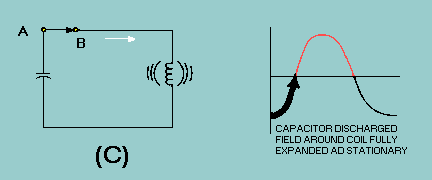Since the capacitor is now completely discharged, the magnetic field surrounding the coil starts to collapse. This induces a voltage in the coil which causes the current to continue flowing in the same direction and charges the capacitor again. This time the capacitor charges to the opposite polarity, view (D). Figure 1-8D. - Capacitor and inductor action in a tank circuit.In view (E), the magnetic field has completely collapsed, and the capacitor has become charged with the opposite polarity. All of the energy is again stored in the capacitor. Figure 1-8E. - Capacitor and inductor action in a tank circuit.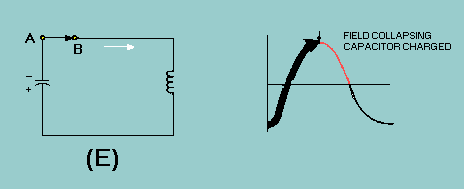In view (F), the capacitor now discharges back through the coil. This discharge current causes the magnetic field to build up again around the coil. Figure 1-8F. - Capacitor and inductor action in a tank circuit.In view (G), the capacitor is completely discharged. The magnetic field is again at maximum. Figure 1-8G. - Capacitor and inductor action in a tank circuit.In view (H), with the capacitor completely discharged, the magnetic field again starts collapsing. The induced voltage from the coil maintains current flowing toward the upper plate of the capacitor. Figure 1-8H. - Capacitor and inductor action in a tank circuit.In view (I), by the time the magnetic field has completely collapsed, the capacitor is again charged with the same polarity as it had in view (A). The energy is again stored in the capacitor, and the cycle is ready to start again. Figure 1-8I. - Capacitor and inductor action in a tank circuit.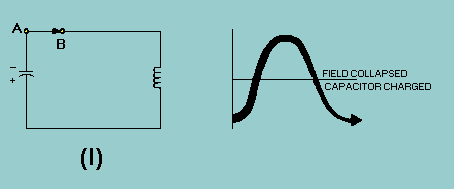The number of times per second that these events in figure 1-8 take place is called NATURAL FREQUENCY or RESONANT FREQUENCY of the circuit. Such a circuit is said to oscillate at its resonant frequency. It might seem that these oscillations could go on forever. You know better, however, if you apply what you have already learned about electric circuits. This circuit, as all others, has some resistance. Even the relatively small resistance of the coil and the connecting wires cause energy to be dissipated in the form of heat (I2R loss). The heat loss in the circuit resistance causes the charge on the capacitor to be less for each subsequent cycle. The result is a DAMPED WAVE, as shown in figure 1-9. The charging and discharging action will continue until all of the energy has been radiated or dissipated as heat. Figure 1-9. - Damped wave.If it were possible to have a circuit with absolutely no resistance, there would be no heat loss, and the oscillations would tend to continue indefinitely. You have already learned that tuned circuits are designed to have very little resistance. Reducing I2R losses is still another reason for having low resistance. A "perfect" tuned circuit would produce the continuous sine wave shown in figure 1-10. Its frequency would be that of the circuit. Figure 1-10. - Sine wave-resonant frequency.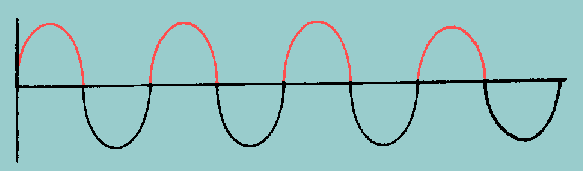Because we don't have perfection, another way of causing a circuit to oscillate indefinitely would be to apply a continuous a.c. or pulsing source to the circuit. If the source is at the resonant frequency of the circuit, the circuit will oscillate as long as the source is applied. The reasons why the circuit in figure 1-8 oscillates at the resonant frequency have to do with the characteristics of resonant circuits. The discussion of parallel resonance will not be as detailed as that for series resonance because the idea of resonance is the same for both circuits. Certain characteristics differ as a result of L and C being in parallel rather than in series. These differences will be emphasized. Q.7 When the capacitor is completely discharged, where is the energy of the tank circuit stored?Q.8 When the magnetic field of the inductor is completely collapsed, where is the energy of the tank circuit stored?Integrated Publishing, Inc. - A (SDVOSB) Service Disabled Veteran Owned Small Business